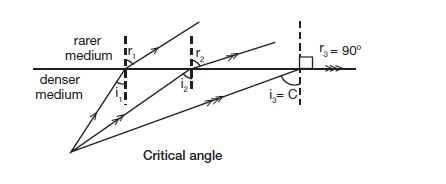# What are the total internal reflection and critical angle?

Last updated on April 14th, 2021 at 05:23 pm

This post is on the total internal reflection and critical angle. Let’s begin.

## What are the total internal reflection and critical angle?

When a ray of light passes from denser to rarer medium, the refracted ray bends away from the normal. Hence, the angle of refraction is greater than the angle of incidence.

Gradually if the angle of incidence increases, the angle of refraction also increases.

Definition of critical angle: At a particular angle of incidence in the denser medium, the refracted ray just grazes the refracting surface making the angle of refraction is equal to 90°. This angle of incidence in the denser medium is called ‘critical angle’,In the diagram above, the angle of incidence i3 is the critical angle that makes the angle of refraction r3=90 degrees.

If the angle of incidence is further increased, the rays do not undergo refraction, it gets reflected into the same optically denser medium. This is known as total internal reflection.

Definition of total internal reflection: When the angle of incidence in the denser medium is greater than the critical angle the light reflected back into the same medium. This phenomenon is called total internal reflection.

In the above diagram, two setups are shown.

The left one is for critical angle set up where i=c, i.e. angle of incidence equals critical angle.

And the setup at the right hand side is showing the total internal reflection setup, where angle i is greater than critical angle c causing the light’s total internal reflection.

See also  Refractive Index (µ) of some common substances | Refractive Index Table

## Why does the light need to pass from denser to rarer medium only (not the reverse one) for Total Internal Reflection to happen?

When light passes from an optically rarer to an optically denser medium, it refracts towards the normal.

But for total internal reflection to happen the ray of light needs to refract away from the normal so that it goes on bending away from the normal (in the rarer medium) as the angle of incidence in denser medium goes on rising.

And finally when the angle of incidence in the denser medium is more than the critical angle then the ray of light reflects totally and comes back totally to the denser medium.

And thus this total internal reflection is only possible if the light passes from denser to rarer medium.

Scroll to top
error: physicsTeacher.in